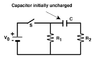# Final current through resistors

• sabak22

## Homework Statement

The diagram below depicts an RC-circuit where C = 3.45 F, = 29.0 , = 28.0 , and = 3.00 Volts

What is the current through immediately after the switch S is closed?
1.03×10-1 A

What is the current through immediately after the switch S is closed?
1.07×10-1 A

What is the current through the capacitor immediately after the switch S is closed?
1.07×10-1 A

What is the current through R1after the switch S has been closed for a very long time? Assume that the battery does not go dead

What is the current through R2 after the switch S has been closed for a very long time? Assume that the battery does not go dead

I= V/R

## The Attempt at a Solution

So I got the first three questions by applying the formula above for the current. As for the current after a long time, i was so sure that the current through a resistor is 0 after a longtime, but when i enter 0A it says Incorrect. Any help?

#### Attachments

•ResistorCapacitorCircuit.gif
2.7 KB · Views: 508